GFG App
Open AppBrowser
Continue

# Search and Insertion in K Dimensional tree

## What is K dimension tree?

A K-D Tree(also called as K-Dimensional Tree) is a binary search tree where data in each node is a K-Dimensional point in space. In short, it is a space partitioning(details below) data structure for organizing points in a K-Dimensional space. A non-leaf node in K-D tree divides the space into two parts, called as half-spaces. Points to the left of this space are represented by the left subtree of that node and points to the right of the space are represented by the right subtree. We will soon be explaining the concept on how the space is divided and tree is formed. For the sake of simplicity, let us understand a 2-D Tree with an example. The root would have an x-aligned plane, the root’s children would both have y-aligned planes, the root’s grandchildren would all have x-aligned planes, and the root’s great-grandchildren would all have y-aligned planes and so on.

Generalization: Let us number the planes as 0, 1, 2, …(K – 1). From the above example, it is quite clear that a point (node) at depth D will have A aligned plane where A is calculated as: A = D mod K

How to determine if a point will lie in the left subtree or in right subtree? If the root node is aligned in planeA, then the left subtree will contain all points whose coordinates in that plane are smaller than that of root node. Similarly, the right subtree will contain all points whose coordinates in that plane are greater-equal to that of root node.

Creation of a 2-D Tree: Consider following points in a 2-D plane: (3, 6), (17, 15), (13, 15), (6, 12), (9, 1), (2, 7), (10, 19)

1. Insert (3, 6): Since tree is empty, make it the root node.
2. Insert (17, 15): Compare it with root node point. Since root node is X-aligned, the X-coordinate value will be compared to determine if it lies in the right subtree or in the left subtree. This point will be Y-aligned.
3. Insert (13, 15): X-value of this point is greater than X-value of point in root node. So, this will lie in the right subtree of (3, 6). Again Compare Y-value of this point with the Y-value of point (17, 15) (Why?). Since, they are equal, this point will lie in the right subtree of (17, 15). This point will be X-aligned.
4. Insert (6, 12): X-value of this point is greater than X-value of point in root node. So, this will lie in the right subtree of (3, 6). Again Compare Y-value of this point with the Y-value of point (17, 15) (Why?). Since, 12 < 15, this point will lie in the left subtree of (17, 15). This point will be X-aligned.
5. Insert (9, 1):Similarly, this point will lie in the right of (6, 12).
6. Insert (2, 7):Similarly, this point will lie in the left of (3, 6).
7. Insert (10, 19): Similarly, this point will lie in the left of (13, 15).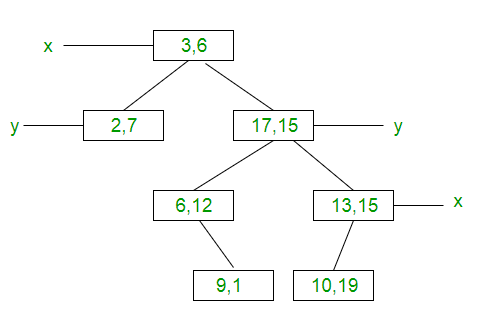How is space partitioned? All 7 points will be plotted in the X-Y plane as follows:

1. Point (3, 6) will divide the space into two parts: Draw line X = 3.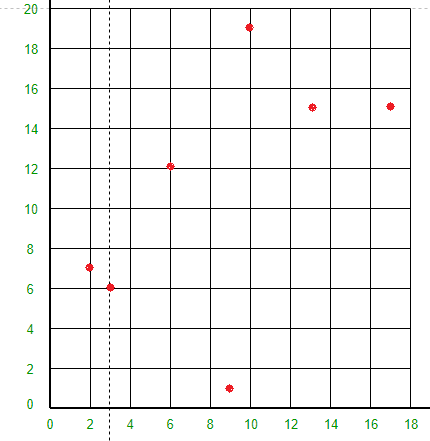2. Point (2, 7) will divide the space to the left of line X = 3 into two parts horizontally. Draw line Y = 7 to the left of line X = 3.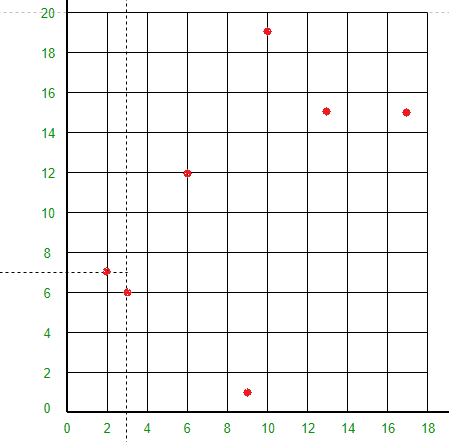3. Point (17, 15) will divide the space to the right of line X = 3 into two parts horizontally. Draw line Y = 15 to the right of line X = 3.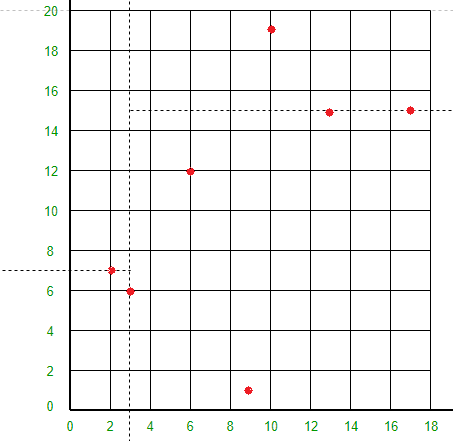• Point (6, 12) will divide the space below line Y = 15 and to the right of line X = 3 into two parts. Draw line X = 6 to the right of line X = 3 and below line Y = 15.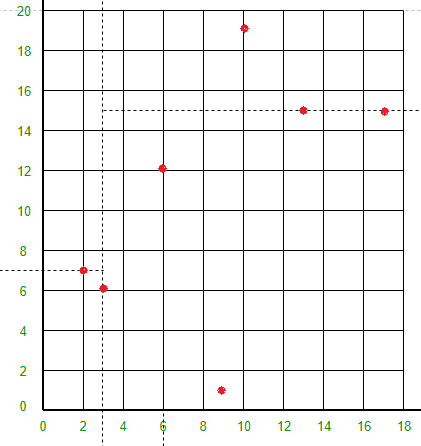• Point (13, 15) will divide the space below line Y = 15 and to the right of line X = 6 into two parts. Draw line X = 13 to the right of line X = 6 and below line Y = 15.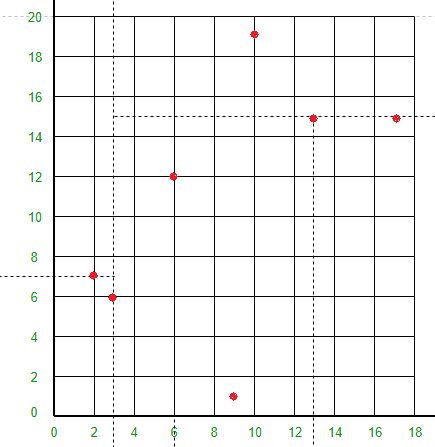• Point (9, 1) will divide the space between lines X = 3, X = 6 and Y = 15 into two parts. Draw line Y = 1 between lines X = 3 and X = 13.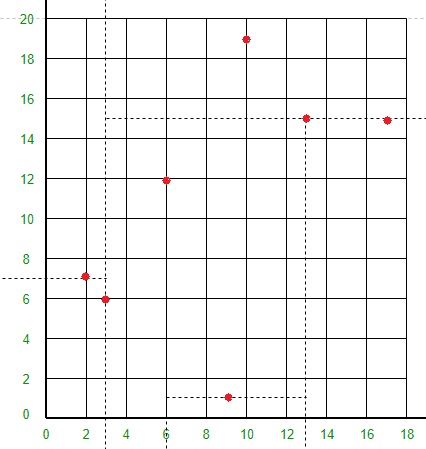• Point (10, 19) will divide the space to the right of line X = 3 and above line Y = 15 into two parts. Draw line Y = 19 to the right of line X = 3 and above line Y = 15.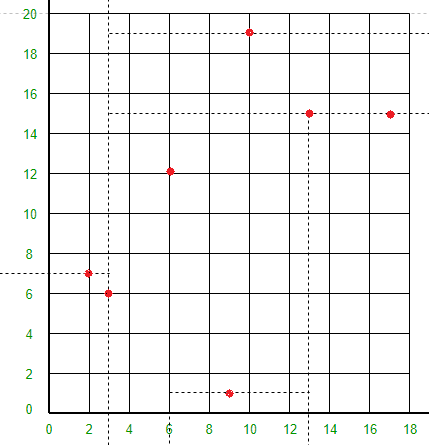Following is C++ implementation of KD Tree basic operations like search, insert and delete.

## C++

 `// A C++ program to demonstrate operations of KD tree` `#include` `using` `namespace` `std;`   `const` `int` `k = 2;`   `// A structure to represent node of kd tree` `struct` `Node` `{` `    ``int` `point[k]; ``// To store k dimensional point` `    ``Node *left, *right;` `};`   `// A method to create a node of K D tree` `struct` `Node* newNode(``int` `arr[])` `{` `    ``struct` `Node* temp = ``new` `Node;`   `    ``for` `(``int` `i=0; ipoint[i] = arr[i];`   `    ``temp->left = temp->right = NULL;` `    ``return` `temp;` `}`   `// Inserts a new node and returns root of modified tree` `// The parameter depth is used to decide axis of comparison` `Node *insertRec(Node *root, ``int` `point[], unsigned depth)` `{` `    ``// Tree is empty?` `    ``if` `(root == NULL)` `       ``return` `newNode(point);`   `    ``// Calculate current dimension (cd) of comparison` `    ``unsigned cd = depth % k;`   `    ``// Compare the new point with root on current dimension 'cd'` `    ``// and decide the left or right subtree` `    ``if` `(point[cd] < (root->point[cd]))` `        ``root->left  = insertRec(root->left, point, depth + 1);` `    ``else` `        ``root->right = insertRec(root->right, point, depth + 1);`   `    ``return` `root;` `}`   `// Function to insert a new point with given point in` `// KD Tree and return new root. It mainly uses above recursive` `// function "insertRec()"` `Node* insert(Node *root, ``int` `point[])` `{` `    ``return` `insertRec(root, point, 0);` `}`   `// A utility method to determine if two Points are same` `// in K Dimensional space` `bool` `arePointsSame(``int` `point1[], ``int` `point2[])` `{` `    ``// Compare individual pointinate values` `    ``for` `(``int` `i = 0; i < k; ++i)` `        ``if` `(point1[i] != point2[i])` `            ``return` `false``;`   `    ``return` `true``;` `}`   `// Searches a Point represented by "point[]" in the K D tree.` `// The parameter depth is used to determine current axis.` `bool` `searchRec(Node* root, ``int` `point[], unsigned depth)` `{` `    ``// Base cases` `    ``if` `(root == NULL)` `        ``return` `false``;` `    ``if` `(arePointsSame(root->point, point))` `        ``return` `true``;`   `    ``// Current dimension is computed using current depth and total` `    ``// dimensions (k)` `    ``unsigned cd = depth % k;`   `    ``// Compare point with root with respect to cd (Current dimension)` `    ``if` `(point[cd] < root->point[cd])` `        ``return` `searchRec(root->left, point, depth + 1);`   `    ``return` `searchRec(root->right, point, depth + 1);` `}`   `// Searches a Point in the K D tree. It mainly uses` `// searchRec()` `bool` `search(Node* root, ``int` `point[])` `{` `    ``// Pass current depth as 0` `    ``return` `searchRec(root, point, 0);` `}`   `// Driver program to test above functions` `int` `main()` `{` `    ``struct` `Node *root = NULL;` `    ``int` `points[][k] = {{3, 6}, {17, 15}, {13, 15}, {6, 12},` `                       ``{9, 1}, {2, 7}, {10, 19}};`   `    ``int` `n = ``sizeof``(points)/``sizeof``(points);`   `    ``for` `(``int` `i=0; i

## Java

 `import` `java.util.*;`   `class` `Node {` `    ``int``[] point;` `    ``Node left, right;`   `    ``public` `Node(``int``[] arr)` `    ``{` `        ``this``.point = arr;` `        ``this``.left = ``this``.right = ``null``;` `    ``}` `}`   `class` `KDtree {` `    ``int` `k = ``2``;`   `    ``public` `Node newNode(``int``[] arr) { ``return` `new` `Node(arr); }`   `    ``public` `Node insertRec(Node root, ``int``[] point, ``int` `depth)` `    ``{` `        ``if` `(root == ``null``) {` `            ``return` `newNode(point);` `        ``}`   `        ``int` `cd = depth % k;` `        ``if` `(point[cd] < root.point[cd]) {` `            ``root.left` `                ``= insertRec(root.left, point, depth + ``1``);` `        ``}` `        ``else` `{` `            ``root.right` `                ``= insertRec(root.right, point, depth + ``1``);` `        ``}`   `        ``return` `root;` `    ``}`   `    ``public` `Node insert(Node root, ``int``[] point)` `    ``{` `        ``return` `insertRec(root, point, ``0``);` `    ``}`   `    ``public` `boolean` `arePointsSame(``int``[] point1, ``int``[] point2)` `    ``{` `        ``for` `(``int` `i = ``0``; i < k; ++i) {` `            ``if` `(point1[i] != point2[i]) {` `                ``return` `false``;` `            ``}` `        ``}` `        ``return` `true``;` `    ``}`   `    ``public` `boolean` `searchRec(Node root, ``int``[] point,` `                             ``int` `depth)` `    ``{` `        ``if` `(root == ``null``) {` `            ``return` `false``;` `        ``}` `        ``if` `(arePointsSame(root.point, point)) {` `            ``return` `true``;` `        ``}`   `        ``int` `cd = depth % k;` `        ``if` `(point[cd] < root.point[cd]) {` `            ``return` `searchRec(root.left, point, depth + ``1``);` `        ``}` `        ``return` `searchRec(root.right, point, depth + ``1``);` `    ``}`   `    ``public` `boolean` `search(Node root, ``int``[] point)` `    ``{` `        ``return` `searchRec(root, point, ``0``);` `    ``}`   `    ``public` `static` `void` `main(String[] args)` `    ``{` `        ``KDtree kdTree = ``new` `KDtree();`   `        ``Node root = ``null``;` `        ``int``[][] points` `            ``= { { ``3``, ``6` `}, { ``17``, ``15` `}, { ``13``, ``15` `}, { ``6``, ``12` `},` `                ``{ ``9``, ``1` `}, { ``2``, ``7` `},   { ``10``, ``19` `} };`   `        ``int` `n = points.length;`   `        ``for` `(``int` `i = ``0``; i < n; i++) {` `            ``root = kdTree.insert(root, points[i]);` `        ``}`   `        ``int``[] point1 = { ``10``, ``19` `};` `        ``System.out.println(kdTree.search(root, point1)` `                               ``? ``"Found"` `                               ``: ``"Not Found"``);`   `        ``int``[] point2 = { ``12``, ``19` `};` `        ``System.out.println(kdTree.search(root, point2)` `                               ``? ``"Found"` `                               ``: ``"Not Found"``);` `    ``}` `}`

## Python3

 `# A Python program to demonstrate operations of KD tree` `import` `sys`   `# Number of dimensions` `k ``=` `2`   `# A structure to represent node of kd tree` `class` `Node:` `    ``def` `__init__(``self``, point):` `        ``self``.point ``=` `point` `        ``self``.left ``=` `None` `        ``self``.right ``=` `None`   `# A method to create a node of K D tree` `def` `newNode(point):` `    ``return` `Node(point)`   `# Inserts a new node and returns root of modified tree` `# The parameter depth is used to decide axis of comparison` `def` `insertRec(root, point, depth):` `    ``# Tree is empty?` `    ``if` `not` `root:` `        ``return` `newNode(point)`   `    ``# Calculate current dimension (cd) of comparison` `    ``cd ``=` `depth ``%` `k`   `    ``# Compare the new point with root on current dimension 'cd'` `    ``# and decide the left or right subtree` `    ``if` `point[cd] < root.point[cd]:` `        ``root.left ``=` `insertRec(root.left, point, depth ``+` `1``)` `    ``else``:` `        ``root.right ``=` `insertRec(root.right, point, depth ``+` `1``)`   `    ``return` `root`   `# Function to insert a new point with given point in` `# KD Tree and return new root. It mainly uses above recursive` `# function "insertRec()"` `def` `insert(root, point):` `    ``return` `insertRec(root, point, ``0``)`   `# A utility method to determine if two Points are same` `# in K Dimensional space` `def` `arePointsSame(point1, point2):` `    ``# Compare individual coordinate values` `    ``for` `i ``in` `range``(k):` `        ``if` `point1[i] !``=` `point2[i]:` `            ``return` `False`   `    ``return` `True`   `# Searches a Point represented by "point[]" in the K D tree.` `# The parameter depth is used to determine current axis.` `def` `searchRec(root, point, depth):` `    ``# Base cases` `    ``if` `not` `root:` `        ``return` `False` `    ``if` `arePointsSame(root.point, point):` `        ``return` `True`   `    ``# Current dimension is computed using current depth and total` `    ``# dimensions (k)` `    ``cd ``=` `depth ``%` `k`   `    ``# Compare point with root with respect to cd (Current dimension)` `    ``if` `point[cd] < root.point[cd]:` `        ``return` `searchRec(root.left, point, depth ``+` `1``)`   `    ``return` `searchRec(root.right, point, depth ``+` `1``)`   `# Searches a Point in the K D tree. It mainly uses` `# searchRec()` `def` `search(root, point):` `    ``# Pass current depth as 0` `    ``return` `searchRec(root, point, ``0``)`   `# Driver program to test above functions` `if` `__name__ ``=``=` `'__main__'``:` `    ``root ``=` `None` `    ``points ``=` `[[``3``, ``6``], [``17``, ``15``], [``13``, ``15``], [``6``, ``12``], [``9``, ``1``], [``2``, ``7``], [``10``, ``19``]]`   `    ``n ``=` `len``(points)`   `    ``for` `i ``in` `range``(n):` `        ``root ``=` `insert(root, points[i])`   `    ``point1 ``=` `[``10``, ``19``]` `    ``if` `search(root, point1):` `        ``print``(``"Found"``)` `    ``else``:` `        ``print``(``"Not Found"``)`   `    ``point2 ``=` `[``12``, ``19``]` `    ``if` `search(root, point2):` `        ``print``(``"Found"``)` `    ``else``:` `        ``print``(``"Not Found"``)` `        `  `# This code is contributed by Prajwal Kandekar`

## C#

 `// C# implementation for the above approach`   `// Importing the System namespace which contains classes used in the program` `using` `System;`   `// Defining the Node class with an integer array to hold the point and references to left and right nodes` `class` `Node {` `  ``public` `int``[] point;` `  ``public` `Node left;` `  ``public` `Node right;`   `  ``// Defining a constructor to initialize the Node object` `  ``public` `Node(``int``[] point) {` `      ``this``.point = point;` `      ``left = ``null``;` `      ``right = ``null``;` `  ``}`   `}`   `// Defining the KdTree class to represent a K-d tree with a specified value of k` `class` `KdTree {` `  ``private` `int` `k;`   `  ``// Defining a constructor to initialize the KdTree object` `  ``public` `KdTree(``int` `k) {` `      ``this``.k = k;` `  ``}`   `  ``// Defining a method to create a new Node object` `  ``public` `Node newNode(``int``[] point) {` `      ``return` `new` `Node(point);` `  ``}`   `  ``// Defining a recursive method to insert a point into the K-d tree` `  ``private` `Node insertRec(Node root, ``int``[] point, ``int` `depth) {` `      ``if` `(root == ``null``) {` `          ``return` `newNode(point);` `      ``}`   `      ``int` `cd = depth % k;`   `      ``if` `(point[cd] < root.point[cd]) {` `          ``root.left = insertRec(root.left, point, depth + 1);` `      ``} ` `      ``else` `{` `          ``root.right = insertRec(root.right, point, depth + 1);` `      ``}`   `      ``return` `root;` `  ``}`   `  ``// Defining a method to insert a point into the K-d tree` `  ``public` `Node insert(Node root, ``int``[] point) {` `      ``return` `insertRec(root, point, 0);` `  ``}`   `  ``// Defining a method to check if two points are the same` `  ``private` `bool` `arePointsSame(``int``[] point1, ``int``[] point2) {` `      ``for` `(``int` `i = 0; i < k; i++) {` `          ``if` `(point1[i] != point2[i]) {` `              ``return` `false``;` `          ``}` `      ``}`   `      ``return` `true``;` `  ``}`   `  ``// Defining a recursive method to search for a point in the K-d tree` `  ``private` `bool` `searchRec(Node root, ``int``[] point, ``int` `depth) {` `      ``if` `(root == ``null``) {` `          ``return` `false``;` `      ``}`   `      ``if` `(arePointsSame(root.point, point)) {` `          ``return` `true``;` `      ``}`   `      ``int` `cd = depth % k;`   `      ``if` `(point[cd] < root.point[cd]) {` `          ``return` `searchRec(root.left, point, depth + 1);` `      ``}`   `      ``return` `searchRec(root.right, point, depth + 1);` `  ``}`   `  ``// Defining a method to search for a point in the K-d tree` `  ``public` `bool` `search(Node root, ``int``[] point) {` `      ``return` `searchRec(root, point, 0);` `  ``}`   `}`   `// Program class to demonstrate the KdTree data structure and its functionality` `class` `Program {` `  `  `  ``static` `void` `Main(``string``[] args) {` `    `  `  ``// Initialize root node as null` `  ``Node root = ``null``;` `    `  `      ``// Define an array of points to be inserted into the tree` `      ``int``[][] points = { ` `          ``new` `int``[] { 3, 6 }, ` `          ``new` `int``[] { 17, 15 }, ` `          ``new` `int``[] { 13, 15 },` `          ``new` `int``[] { 6, 12 }, ` `          ``new` `int``[] { 9, 1 }, ` `          ``new` `int``[] { 2, 7 },` `          ``new` `int``[] { 10, 19 } ` `      ``};`   `      ``// Get the length of the points array` `      ``int` `n = points.Length;`   `      ``// Create a new KdTree object with k=2` `      ``KdTree tree = ``new` `KdTree(2);`   `      ``// Insert all the points into the tree and update the root node` `      ``for` `(``int` `i = 0; i < n; i++) {` `          ``root = tree.insert(root, points[i]);` `      ``}`   `      ``// Define a point to search for in the tree` `      ``int``[] point1 = { 10, 19 };`   `      ``// Search for the point in the tree and print the result` `      ``if` `(tree.search(root, point1)) {` `          ``Console.WriteLine(``"Found"``);` `      ``} ``else` `{` `          ``Console.WriteLine(``"Not Found"``);` `      ``}`   `      ``// Define another point to search for in the tree` `      ``int``[] point2 = { 12, 19 };`   `      ``// Search for the point in the tree and print the result` `      ``if` `(tree.search(root, point2)) {` `          ``Console.WriteLine(``"Found"``);` `      ``} ``else` `{` `          ``Console.WriteLine(``"Not Found"``);` `      ``}` `  ``}` `}`   `// This code is contributed by Amit Mangal.`

## Javascript

 `// JavaScript code for the above approach`   `// Number of dimensions` `const k = 2;`   `// A structure to represent node of kd tree` `class Node {` `  ``constructor(point) {` `    ``this``.point = point;` `    ``this``.left = ``null``;` `    ``this``.right = ``null``;` `  ``}` `}`   `// A method to create a node of K D tree` `function` `newNode(point) {` `  ``return` `new` `Node(point);` `}`   `// Inserts a new node and returns root of modified tree` `// The parameter depth is used to decide axis of comparison` `function` `insertRec(root, point, depth) {` `  ``// Tree is empty?` `  ``if` `(!root) {` `      ``return` `newNode(point);` `  ``}`   `  ``// Calculate current dimension (cd) of comparison` `  ``const cd = depth % k;`   `  ``// Compare the new point with root on current dimension 'cd'` `  ``// and decide the left or right subtree` `  ``if` `(point[cd] < root.point[cd]) {` `      ``root.left = insertRec(root.left, point, depth + 1);` `  ``} ``else` `{` `      ``root.right = insertRec(root.right, point, depth + 1);` `  ``}`   `  ``return` `root;` `}`   `// Function to insert a new point with given point in` `// KD Tree and return new root. It mainly uses above recursive` `// function "insertRec()"` `function` `insert(root, point) {` `    ``return` `insertRec(root, point, 0);` `}`   `// A utility method to determine if two Points are same` `// in K Dimensional space` `function` `arePointsSame(point1, point2) {`   `    ``// Compare individual coordinate values` `    ``for` `(let i = 0; i < k; i++) {` `      ``if` `(point1[i] !== point2[i]) {` `          ``return` `false``;` `    ``}` `  ``}`   `  ``return` `true``;` `}`   `// Searches a Point represented by "point[]" in the K D tree.` `// The parameter depth is used to determine current axis.` `function` `searchRec(root, point, depth) {`   `  ``// Base cases` `  ``if` `(!root) {` `      ``return` `false``;` `  ``}` `  `  `  ``if` `(arePointsSame(root.point, point)) {` `      ``return` `true``;` `  ``}`   `  ``// Current dimension is computed using current depth and total` `  ``// dimensions (k)` `  ``const cd = depth % k;`   `  ``// Compare point with root with respect to cd (Current dimension)` `  ``if` `(point[cd] < root.point[cd]) {` `      ``return` `searchRec(root.left, point, depth + 1);` `  ``}`   `  ``return` `searchRec(root.right, point, depth + 1);` `}`   `// Searches a Point in the K D tree. It mainly uses` `// searchRec()` `function` `search(root, point) {`   `// Pass current depth as 0` `    ``return` `searchRec(root, point, 0);` `}`   `// Driver program to test above functions` `let root = ``null``;` `const points = [` `  ``[3, 6],` `  ``[17, 15],` `  ``[13, 15],` `  ``[6, 12],` `  ``[9, 1],` `  ``[2, 7],` `  ``[10, 19],` `];`   `const n = points.length;`   `for` `(let i = 0; i < n; i++) {` `    ``root = insert(root, points[i]);` `}`   `const point1 = [10, 19];`   `if` `(search(root, point1)) {` `    ``console.log(``"Found"``);` `} `   `else` `{` `    ``console.log(``"Not Found"``);` `}`   `const point2 = [12, 19];`   `if` `(search(root, point2)) {` `    ``console.log(``"Found"``);` `} `   `else` `{` `    ``console.log(``"Not Found"``);` `}`   `// This code is contributed by Amit Mangal`

Output:

```Found
```

Time Complexity: O(n)

Auxiliary Space: O(n)

Refer below articles for find minimum and delete operations.

#### Advantages of K Dimension tree –

K-d trees have several advantages as a data structure:

• Efficient search: K-d trees are effective in searching for points in a k-dimensional space, such as in nearest neighbor search or range search.
• Dimensionality reduction: K-d trees can be used to reduce the dimensionality of the problem, allowing for faster search times and reducing the memory requirements of the data structure.
• Versatility: K-d trees can be used for a wide range of applications, such as in data mining, computer graphics, and scientific computing.
• Balance: K-d trees are self-balancing, which ensures that the tree remains efficient even when data is inserted or removed.
• Incremental construction: K-d trees can be incrementally constructed, which means that data can be added or removed from the structure without having to rebuild the entire tree.
• Easy to implement: K-d trees are relatively easy to implement and can be implemented in a variety of programming languages.